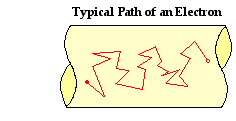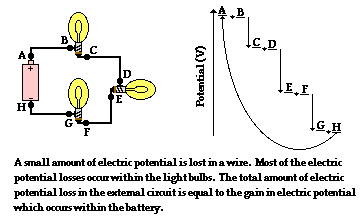Electric Circuits - Lesson 3 - Electrical Resistance

# Journey of a Typical Electron

As mentioned in Lesson 1, an electrochemical cell supplies energy to move a charge from its low energy, low potential terminal to the high energy, high potential terminal. In this sense, the cell supplies the energy to establish an electric potential difference across the two ends of the external circuit. Charge will then flow through the external circuit in the same manner that water will flow from an elevated position to a low position. It is the difference in potential that causes this flow.

In the wires of electric circuits, an electron is the actual charge carrier. As mentioned in Lesson 2, an electron's path through the external circuit is far from being a straight path. Anelectron's journey through a circuit can be described as a zigzag path that results from countless collisions with the atoms of the conducting wire. Each collision results in the alteration of the path, thus leading to a zigzag type motion. While the electric potential difference across the two ends of a circuit encourages the flow of charge, it is the collisions of charge carriers with atoms of the wire that discourages the flow of charge. Different types of atoms offer a different degree of hindrance to the flow of the charge carriers that pass through it.

In all cases, the collisions of charge carriers in an electric circuit with the conducting elements of that circuit result in a loss of energy. While most the electrical energy possessed by a charge carrier is lost when it passes through an electrical device (often referred to as the load), even the wires of the circuit themselves act to remove energy from a charge. It is because of this energy loss in the load and in the wires themselves that the electric potential of a charge carrier is decreased as it traverses the external circuit. The electric energy supplied by the electrochemical cells becomes entirely used up in the external circuit.

In an electric circuit with several electrical devices, there may be multiple stepwise losses of electric potential as the charge traverses the circuit. There are several ways that multiple devices can be wired within a circuit; this will be the topic of Lesson 4. Regardless of the way in which the devices are wired, the total loss of electric potential of a single charge as it passes through the external circuit is equal to the gain in electric potential that it experiences in the battery. As depicted in the diagram below, a charge carrier traversing the external circuit from A to H passes through three different light bulbs. Each light bulb results in a loss of electric potential for the charge. This loss in electric potential corresponds to a loss of energy as the electrical energy is transformed by the light bulb into light energy and thermal energy. In addition to the changes in electric potential and electric energy that occur in the light bulbs, there is also a smaller amount of electric potential loss in the wires that connect the light bulbs. This small amount of loss in electric potential also corresponds to a small loss of energy as the electrical energy is transformed into thermal energy. The wires get hot - not as hot as the light bulb, but still measurably hot.So the journey of an electron through an external circuit involves a long and slow zigzag path that is characterized by several successive losses in electric potential. Each loss of potential is referred to as a voltage drop. Accompanying this voltage drop is a voltage boost occurring within the internal circuit - for instance, within the electrochemical cell. In the next part of Lesson 3 we will look extensively at resistance - the cause for these voltage drops.

Next Section: